Biot Savart Law - Electrical Engineering Gate

# Electrical Engineering Gate

Online Electrical Engineering Study Site

## Thursday, October 16, 2014

Introduction

A useful law that provides a method to calculate the magnetic field produced by an arbitrary current distribution

First discovered by Jean-Baptiste Biot and Félix Savart in the beginning of 19th century

Equation

### (Electric currents (along closed curve

The Biot–Savart law is used for computing the resultant magnetic field B at position r generated by a steady current I (for example due to a wire): a continual flow of chargeswhich is constant in time and the charge neither accumulates nor depletes at any point. The law is a physical example of a line integral: evaluated over the path C the electric currents flow. The equation in SI units is

where r is the full displacement vector from the wire element to the point at which the field is being computed and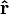$\mathbf{\hat{r}}$ is the unit vector of r. Using$\mathbf{\hat{r}}$, the equation can be equivalently written as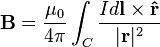$\mathbf{B} = \frac{\mu_0}{4\pi}\int_C \frac{I d\mathbf{l} \times \mathbf{\hat r}}{|\mathbf{r}|^2}$
where dl is a vector whose magnitude is the length of the differential element of the wire, in the direction of conventional current, and μ0 is the magnetic constant. The symbols in boldface denote vector quantities
The integral is usually around a closed curve, since electric currents can only flow around closed paths. An infinitely long wire (as used in the definition of the SI unit of electric current - the Ampere) is a counter-example
To apply the equation, the point in space where the magnetic field is to be calculated is arbitrarily chosen. Holding that point fixed, the line integral over the path of the electric currents is calculated to find the total magnetic field at that point. The application of this law implicitly relies on the superposition principle for magnetic fields, i.e. the fact that the magnetic field is a vector sum of the field created by each infinitesimal section of the wire individually

### (Electric currents (throughout conductor volume

The formulations given above work well when the current can be approximated as running through an infinitely-narrow wire. If the current has some thickness, the proper formulation of the Biot–Savart law (again in SI units) is

or equivalently$\mathbf{B} = \frac{\mu_0}{4\pi}\iiint_V \ \frac{(\mathbf{J}\, dV) \times \mathbf{\hat r}}{r^2}$
.where dV is the differential element of volume and J is the current density vector in that volume
In this case the integral is over the volume of the conductor
The Biot–Savart law is fundamental to magnetostatics, playing a similar role to Coulomb's law in electrostatics. When magnetostatics does not apply, the Biot–Savart law should be replaced by Jefimenko's equations

### Constant uniform current

In the special case of a steady constant current I, the magnetic field B is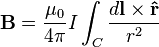$\mathbf B = \frac{\mu_0}{4\pi} I \int_C \frac{d\mathbf l \times \mathbf{\hat r}}{r^2}$
i.e. the current can be taken out of the integral

### Point charge at constant velocity

In the case of a point charged particle q moving at a constant velocity vMaxwell's equations give the following expression for the electric field and magnetic field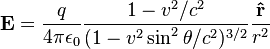$\mathbf{E} = \frac{q}{4\pi \epsilon_0} \frac{1-v^2/c^2}{(1-v^2\sin^2\theta/c^2)^{3/2}}\frac{\mathbf{\hat r}}{r^2}$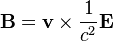$\mathbf{B} = \mathbf{v} \times \frac{1}{c^2} \mathbf{E}$
where  is the vector pointing from the current (non-retarded) position of the particle to the point at which the field is being measured, and θ is the angle between v and r
When v2 ≪ c2, the electric field and magnetic field can be approximated as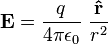$\mathbf{E} =\frac{q}{4\pi\epsilon_0}\ \frac{\mathbf{\hat r}}{r^2}$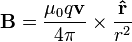$\mathbf{B} =\frac{\mu_0 q \mathbf{v}}{4\pi} \times \frac{\mathbf{\hat r}}{r^2}$
These equations are called the "Biot–Savart law for a point charge" due to its closely analogous form to the "standard" Biot–Savart law given previously. These equations were first derived by Oliver Heaviside in 1888